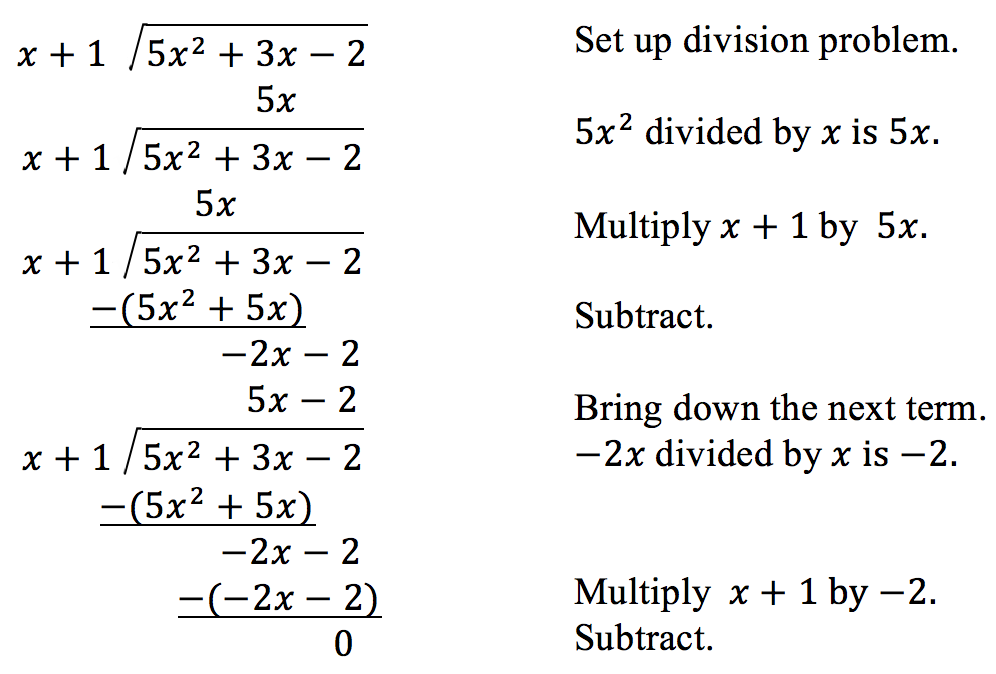## Polynomial Division Unit Test

Posted: September 25, 2020 in Algebra 2

Complete all assignments, quizzes in the polynomial division unit before taking the unit test.

## Polynomial Remainder Theorem

Posted: September 24, 2020 in Algebra 2
 Learn Intro to the Polynomial Remainder Theorem Remainder theorem: finding remainder from equation Remainder theorem examples Remainder theorem: checking factors Remainder theorem: finding coefficients Proof of the Polynomial Remainder Theorem

## 2.1 Derivative and the Tangent Line Problem

Posted: September 24, 2020 in AP Calculus
• Find the slope of the tangent line to a curve at a point.
• Use the limit definition to find the derivative of a function.

 HOMEWORK Pg 131 2, 13-25 odd, 29, 32, 33,35,37-42,51,54,63,65,71,73,79,81,88-90

## Dividing polynomials by linear factors

Posted: September 23, 2020 in Algebra 2
Learn
Dividing polynomials by linear expressions
Dividing polynomials by linear expressions: missing term
Factoring using polynomial division
Factoring using polynomial division: missing term

## Limits and Properties

Posted: September 23, 2020 in AP Calculus

## Review Practice for Limits and Their Properties

Posted: September 22, 2020 in AP Calculus

Review Practice for Limits and Their Properties

• Pg 120 1-17

## Dividing quadratics by linear factors

Posted: September 22, 2020 in Algebra 2

##Learn
Intro to long division of polynomials
Dividing quadratics by linear expressions (no remainders)
Dividing quadratics by linear expressions with remainders
Dividing quadratics by linear expressions with remainders: missing x-term

Practice
Divide quadratics by linear expressions (no remainders)
Divide quadratics by linear expressions (with remainders)

## Polynomial division introduction

Posted: September 21, 2020 in Algebra 2

Math is Fun link to assist you with polynomial division the next few days (Links to an external site.)

Polynomial division introduction
Dividing polynomials by x (no remainders)

Divide polynomials by x (with remainders)
Practice
Divide polynomials by x (no remainders)
Divide polynomials by x (with remainders)

## Quiz in WebAssign

Posted: September 21, 2020 in AP Calculus

QQuiz over  1.4-1.6 in webAssign

## 1.6 Limits at Infinity

Posted: September 18, 2020 in AP Calculus